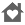# Maths SolutionsEveryone
15
Maths Solutions is an educational app aimed primarily at 2nd level mathematics students while also covering a number of 3rd level topics.

The app functions as both a reference containing a large collection of useful formula and as a formula calculator for computing fully worked solutions to common exam questions.

Areas covered:

Algebra
* Quadratic equations
* Matrices and systems of equations
* Indices and logarithms
* Factorials
* Permutations and combinations
* De Moivre's theorem
* Binomial theorem

Length and Area
* Triangle
* Parallelogram
* Trapezium
* Circle
* Arc and Sector

Surface Area and Volume
* Cylinder
* Frustum
* Cone
* Prism and pyramid
* Sphere

Sequences and Series
* Arithmetic sequence or series
* Geometric sequence or series

Area Approximations
* Trapezoidal rule
* Simpson's rule

Differentiation
* Common Derivatives and Rules
* Common function derivatives

Integration
* Common function integrals
* Integration rules

Statistics
* Mean and standard deviation
* Binomial distribution
* Poisson distribution
* Standard normal distribution tables
* Normal Gaussian distribution
* Student's t-distribution
* Chi-squared distribution
* Confidence intervals
* Hypothesis testing formulae
* One-sample hypothesis testing

Trigonometry
* Unit circle
* Triangle
* Right-angled triangle
* Compound and double angle formulae
* Products to/from sums and differences

Geometry
* Vector notation
* Vector operations
* Co-ordinate geometry of line
* Co-ordinate geometry of circle

Economics and Finance
* Economics formulae
* Finance formulae
* Compound interest
* Annual percentage rate

Chemistry
* Periodic table of elements
* Principal shells and subshells
* Constants
* Boyle's and Charles' Laws
* Combined gas law
* Ideal gas law
* pH calculations

Mathematics is universal, but Irish Leaving Certificate students will find the app particularly relevant.
Read more
Collapse

Review Policy
4.5
15 total
5
4
3
2
1
Loading...

## What's New

Version 1.0.3 - 31/05/2012
* Full support for Holo Theme

Version 1.0.1 - 02/02/2012
Minor update.
* Improved mathematical typesetting for most sections
* Expanded Geometric Sequences and Series section
* Fixed a rounding error in Combinations and Permutations section

Version 1.0 - 29/12/2011
* First release
Read more
Collapse

## Additional Information

Eligible for Family LibraryEligible if bought after 7/2/2016. Learn More
Updated
June 25, 2013
Size
1.5M
Installs
100+
Current Version
1.0.3
Requires Android
2.2 and up
Content Rating
Everyone
Permissions
Offered By
Eoin O'Callaghan
Developer
44 The Village, Newbridge, Kildare
©2019 GoogleSite Terms of ServicePrivacyDevelopersArtistsAbout Google|Location: United StatesLanguage: English (United States)
By purchasing this item, you are transacting with Google Payments and agreeing to the Google Payments Terms of Service and Privacy Notice.